2022: SklogWiki celebrates 15 years on-line

Ballone-Pastore-Galli-Gazzillo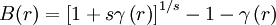$B(r)=\left[ 1+s\gamma \left( r\right) \right] ^{1/s}-1-\gamma \left(r\right)$
where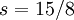$s=15/8$. It has its origins in the Martynov-Sarkisov closure (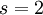$s=2$). The value of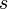$s$ can be determined by a self-consistency condition. Notice that for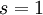$s=1$ the BPGG approximation reduces to the hyper-netted chain closure.